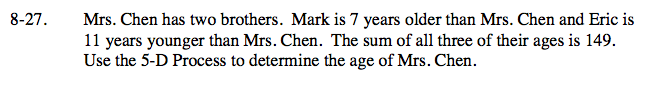### Home > CC2 > Chapter 8 > Lesson 8.1.2 > Problem8-27

8-27.

Mrs. Chen has two brothers. Mark is 7 years older than Mrs. Chen and Eric is 11 years younger than Mrs. Chen. The sum of all three of their ages is 149. Use the 5-D Process to determine the age of Mrs. Chen. Homework Help ✎What are the equations you can write to relate the ages of Mrs. Chen, Mark, and Eric? How can you use these equations to find Mrs. Chen's age?

If C = Mrs. Chen's age, M = Mark's age, and E = Eric's age one of the equations is C + M + E = 149.
Can you find the others?

Mrs. Chen is 51 years old.